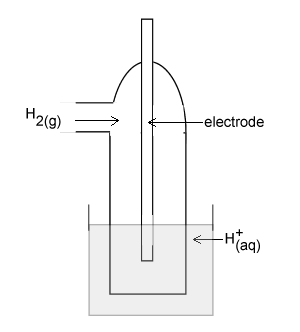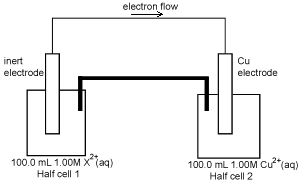Galvanic cells Past exam question The diagram on the right represents a H+(aq)/H2(g) half cell for the reaction 2H+(aq) + 2e- => H2(g). Identify an appropriate material for the electrode.For this half cell to be a standard half cell, state the; - temperature at which it must operate; Solution - required pH of the solution of H+(aq) ions. SolutionA galvanic cell consists of the following  half cells which have been set up under standard conditions. - Half cell 1- the H+(aq)/H2(g) shown above. - Half cell 2- a cadmium (Cd) electrode in a solution containing Cd2+(aq)   After some time, the pH in half cell 1has increased. Use this information to identify the species in this galvanic cell which is the stronger reductant and explain how you reached this conclusion. Solution A second galvanic cell is shown on the right. After discharging 2654 C of electricity, the concentration of the X2+(aq) in solution in half cell 1 was found to be 0.725 M. The volume of the solutions in the two half cells had not changed.Calculate the amount, in mol, of X2+(aq) that reacted in the half cell1 SolutionCalculate the ratio of n(X2+) reacted to n(e-) that passed through the cell. That is, calculate  n(X2+) reacted: n(e-) Solution State the oxidation state of the product of the half cell 1 Write an equation for the reaction that occurred at the electrode of half cell 1 Solution